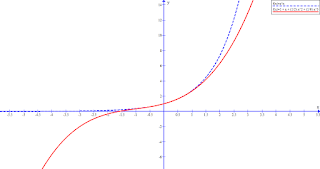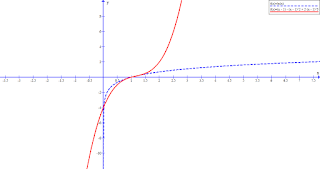## Thursday, August 30, 2018

### The McLauren series and Taylor series (approximating complicated functions with simple polynomials)

The McLauren series

Imagine you had a function $f(x)$ that you knew was a polynomial, but whose details were unknown and you could only apply operations to the function without being able to read it. How could you find the coefficients of this polynomial? We know that for coefficients $a_i$:

$f(x) = a_0 + a_1 x + a_2 x^2 + a_3 x^3 + a_4 x^4 + ...$

If we find $f(0)$ then we can find $a_0$.

$f(0) = a_0 + a_1 0 + a_2 0^2 + a_3 0^3 + a_4 0^4 + ... = a_0 + 0 + 0 + ... = a_0$

That was easy, but how can we find $a_1$? We need an operation that gets rid of $a_0$ and also the $x$ in the term $a_1 x$. That operation turns out to be differentiation with respect to $x$:

$f'(x) = a_1 + 2 a_2 x + 3 a_3 x^2 + 4 a_4 x^3 + ...$

Great! Now we can find $a_1$ by replacing $x$ with 0:

$f'(0) = a_1 + 2 a_2 0 + 3 a_3 0^2 + 4 a_4 0^3 + ... = a_1$

We can find $a_2$ by repeating these two steps:

$f''(x) = 2 a_2 + 2 \cdot 3 a_3 x + 3 \cdot 4 a_4 x^2 + ...$
$f''(0) = 2 a_2 + 2 \cdot 3 a_3 0 + 3 \cdot 4 a_4 0^2 + ... = 2 a_2$

What we found is twice of $a_2$ which means that we need to divide by 2 to get $a_2$. The differentiation operation is multiplying constants by each coefficient and the constants get bigger and bigger the more times we apply differentiation. You might notice that what's happening is that $a_0$ was multiplied by 1, $a_1$ was also multiplied by 1, $a_2$ has been multiplied by 2, $a_3$ will be multiplied by 6, $a_4$ by 24, and so on. These are factorials, sequences of whole numbers multiplied together ($3! = 1 \times 2 \times 3 = 6$). Which means that we need to divide by the next factorial after each round of differentiation and substitution by zero.

In general we can find the $i$th coefficient in an unknown polynomial function by doing the following:

$a_i = \frac{f^i(0)}{i!}$

That's great. Now to test it. Let's see if a complex function is actually a polynomial in disguise. Take something simple such as $f(x) = e^x$. This doesn't look like a polynomial, but it may be represented by a polynomial with an infinite number of terms. Let's find what are the coefficients of the hidden polynomial in $e^x$.

$f(x) = e^x = a_0 + a_1 x + a_2 x^2 + a_3 x^3 + a_4 x^4 + ...$
$\frac{f(0)}{0!} = \frac{e^0}{1} = a_0$
$\frac{f(0)}{0!} = 1$

OK, so $a_0$ is 1. Let's find the rest of the coefficients.

$a_1 = \frac{f'(0)}{1!} = \frac{e^0}{1} = 1$
$a_2 = \frac{f''(0)}{2!} = \frac{e^0}{2} = \frac{1}{2}$
$a_3 = \frac{f'''(0)}{3!} = \frac{e^0}{6} = \frac{1}{6}$
$...$

So the first few terms of the polynomial hidden within $e^x$ are:
$f(x) = 1 + x + \frac{1}{2} x^2 + \frac{1}{6} x^3 + ...$

Does this partial polynomial look anything like $e^x$ when plotted as a graph?Pretty good within a boundary! Note how the curve has a perfect fit at $x = 0$ and that it gets worse as we move away from there. Adding more terms to the polynomial will enlarge the area around $x = 0$ that is close to the curve but it will always be radiating out from there.

Let's try for $f(x) = cos(x)$ now.

$a_0 = \frac{f(0)}{0!} = \frac{cos(0)}{1} = 1$
$a_1 = \frac{f'(0)}{1!} = \frac{-sin(0)}{1} = 0$
$a_2 = \frac{f''(0)}{2!} = \frac{-cos(0)}{2} = -\frac{1}{2}$
$a_3 = \frac{f'''(0)}{3!} = \frac{sin(0)}{6} = 0$
$...$

So the first few terms of the polynomial hidden within $cos(x)$ is
$f(x) = 1 - \frac{1}{2} x^2 + ...$The Taylor series

As you can see, this "polynomialisation" of functions is a neat way to approximate a function we might not know how to implement exactly but know how to differentiate and how to find its value when $x$ is 0. But what if we don't know what $f(0)$ is such as in $ln(x)$ or $\frac{1}{x}$? A slight modification to our method allows us to use any value of $x$ and not just 0. Let's call this value $b$. By slightly modifying the polynomial we expect to be hiding inside the function, we can make the polynomial act the same way when $f(b)$ is used instead of $f(0)$:

$f(x) = a_0 + a_1 (x - b) + a_2 (x - b)^2 + a_3 (x - b)^3 + a_4 (x - b)^4 + ...$
$f(b) = a_0 + a_1 (b - b) + a_2 (b - b)^2 + a_3 (b - b)^3 + a_4 (b - b)^4 + ...$
$f(b) = a_0$

$f'(x) = a_1 + 2 a_2 (x - b) + 3 a_3 (x - b)^2 + 4 a_4 (x - b)^3 + ...$
$f'(b) = a_1$

$a_i = \frac{f^i(b)}{i!}$

The catch here is that we are now finding coefficients to the polynomial $a_0 + a_1 (x - b) + a_2 (x - b)^2 + ...$ and not of $a_0 + a_1 x + a_2 x^2 + ...$, but that's OK. Let's try this on $ln(x)$ with $b = 1$.

$a_0 = \frac{f(1)}{0!} = \frac{ln(1)}{1} = 0$
$a_1 = \frac{f'(1)}{1!} = \frac{\frac{1}{1}}{1} = 1$
$a_2 = \frac{f''(1)}{2!} = \frac{-\frac{1}{1^2}}{1} = -1$
$a_3 = \frac{f'''(1)}{3!} = \frac{\frac{2}{1^3}}{1} = 2$
$...$

So the first few terms of the polynomial hidden within $ln(x)$ is
$f(x) = (x - 1) - (x - 1)^2 + 2 (x - 1)^3 + ...$Adding more terms will approximate the original function better and better but what if we didn't have to? Remember how I said in the previous section that the polynomial approximates the original function best close to $x = 0$. Well now we can approximate it best around any point $b$ and not just around 0. This means that if our function has multiple known values, such as $cos(x)$ which is known to be 1 at $x = 0$, 0 at $x = \frac{\pi}{2}$, -1 at $x = \pi$, etc., then we can use several short polynomials centered at different points in the function instead of one large polynomial that approximates it well over a large interval. Let's try approximating $cos(x)$ around $x = \pi$, which means that we'll set $b$ to $\pi$.

$a_0 = \frac{f(\pi)}{0!} = \frac{cos(\pi)}{1} = -1$
$a_1 = \frac{f'(\pi)}{1!} = \frac{-sin(\pi)}{1} = 0$
$a_2 = \frac{f''(\pi)}{2!} = \frac{-cos(\pi)}{2} = \frac{1}{2}$
$a_3 = \frac{f'''(\pi)}{3!} = \frac{sin(\pi)}{6} = 0$
$...$

So the first few terms of the polynomial hidden within $cos(x)$ which best approximates the area around $x = \pi$ is
$f(x) = -1 + \frac{1}{2} (x - \pi)^2 + ...$This is useful when implementing mathematical functions on a computer. You keep several simple polynomials defined at different points in the domain of the function and then pick the closest one to the $x$ you need to evaluate. You can then compute an approximation that isn't too bad without requiring a lot of computational time.